Sri Yantra Definition

This page describes the basic characteristics that describe the Sri Yantra geometry.

 The Sri Yantra is composed of nine interlocking triangles. Four triangles pointing up (U1, U2, U3, U4) and five triangles pointing down (D1, D2, D3, D4, D5). Only triangles (U3, D3, D4) are completely enclosed by another triangle.

1.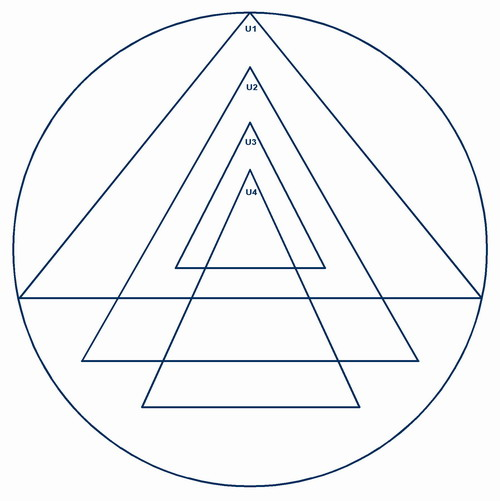1. The two biggest triangles touch the outer cirlce on all three points.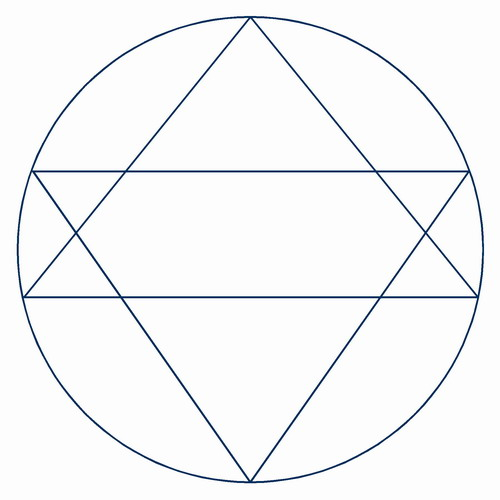1. Except for the two biggest triangles, all apexes touch the base of another triangle.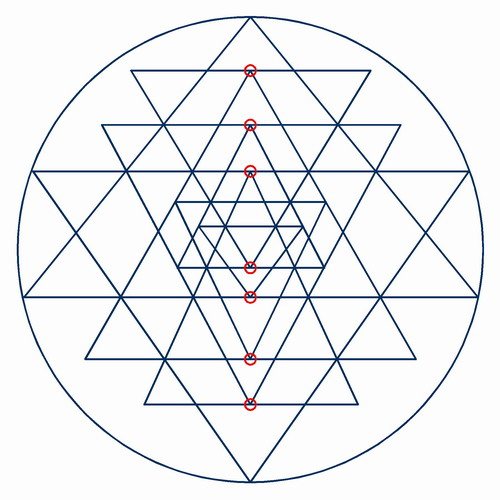1. There are 33 intersections resulting from three lines meeting at a point.
Note: Some configurations can have 4 additional such intersections (U4 and D5 corners touch the outside circle) for a total of 37.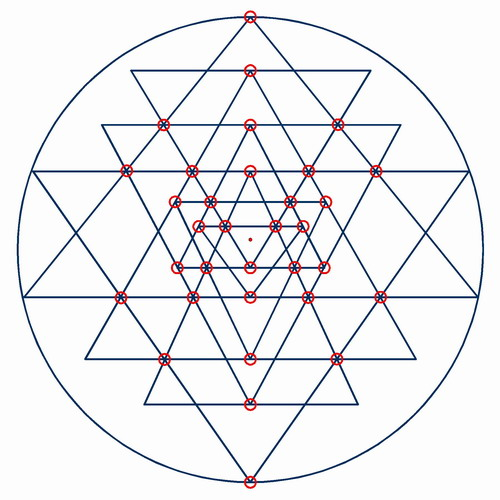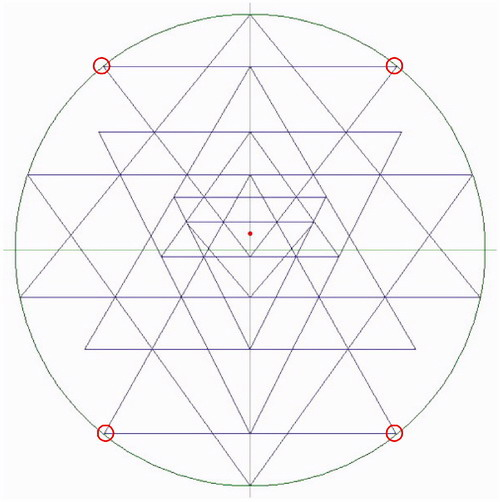1. There are 24 intersections resulting from two lines crossing.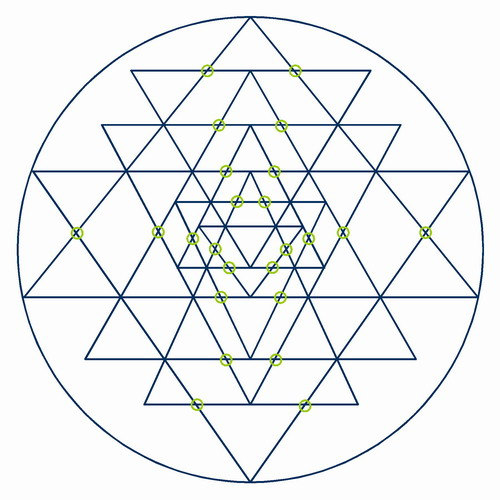1. A total of 43 triangles are created from the overlapping of the nine original triangles.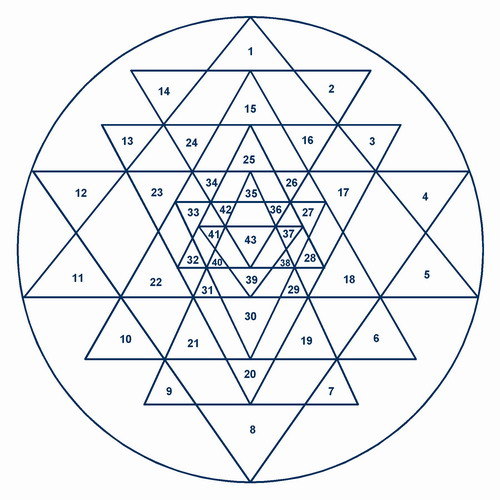1. The bindu point is located at the geometric center of the innermost triangle.